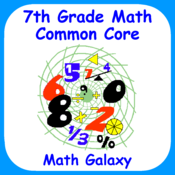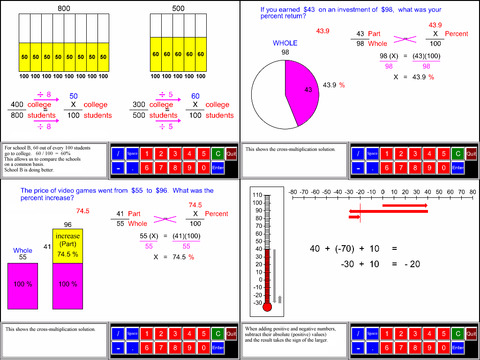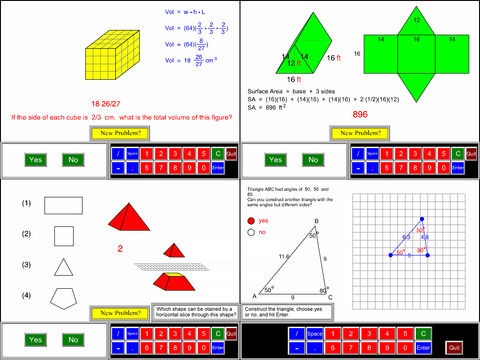# 7th Grade Math Common Core - Lumos Educational App Store41
Price -\$5.99
\$5.99

#### DESCRIPTION:

In seventh grade math the emphasis is on: (1) ratios & proportions, (2) rational and negative numbers, (3) linear equations, (4) scale drawings, area, surface area and volume, and (6) statistical thinking.Math Galaxy's Seventh Grade Common Core Math covers all of the Common Core math standards for seventh grade as listed below.The concepts are presented in bite-size pieces with an unlimited supply of practice problems, with numbers chosen randomly.Illustrations are used throughout to reinforce the concepts.You can be guided through problems step-by-step or just give final answers.Cer

#### OVERVIEW:

7th Grade Math Common Core is a free educational mobile app By Math Galaxy.It helps students in grades 7 practice the following standards 7.EE.1,7.EE.2,7.EE.3.

This page not only allows students and teachers download 7th Grade Math Common Core but also find engaging Sample Questions, Videos, Pins, Worksheets, Books related to the following topics.

1. 7.EE.1 : Apply properties of operations as strategies to add, subtract, factor, and expand linear expressions with rational coefficients..

2. 7.EE.2 : Use properties of operations to generate equivalent expressions. Understand that rewriting an expression in different forms in a problem context can shed light on the problem and how the quantities in it are related. For example, a + 0.05a = 1.05a means that .

3. 7.EE.3 : Solve multi-step real-life and mathematical problems posed with positive and negative rational numbers in any form (whole numbers, fractions, and decimals), using tools strategically. Apply properties of operations as strategies to calculate with numbers in any form; convert between forms as appropriate; and assess the reasonableness of answers using mental computation and estimation strategies. For example: If a woman making \$25 an hour gets a 10% raise, she will make an additional 1/10 of her salary an hour, or \$2.50, for a new salary of \$27.50. If you want to place a towel bar 9 3/4 inches long in the center of a door that is 27 1/2 inches wide, you will need to place the bar about 9 inches from each edge; this estimate can be used as a check on the exact computation..

7

#### STANDARDS:

7.EE.1
7.EE.2
7.EE.3
7.EE.4
7.G.1
7.G.2
7.G.3
7.G.4
7.G.5
7.G.6
7.NS.1
7.NS.2
7.NS.3
7.RP.1
7.RP.2
7.RP.3
7.SP.1
7.SP.2
7.SP.3
7.SP.4
7.SP.5
7.SP.6
7.SP.7
7.SP.8

Developer: Math Galaxy

Software Version: 1.1

Category: Education

Release Date: Sep 14, 2015### RELATED APPSEdSearch WebSearch